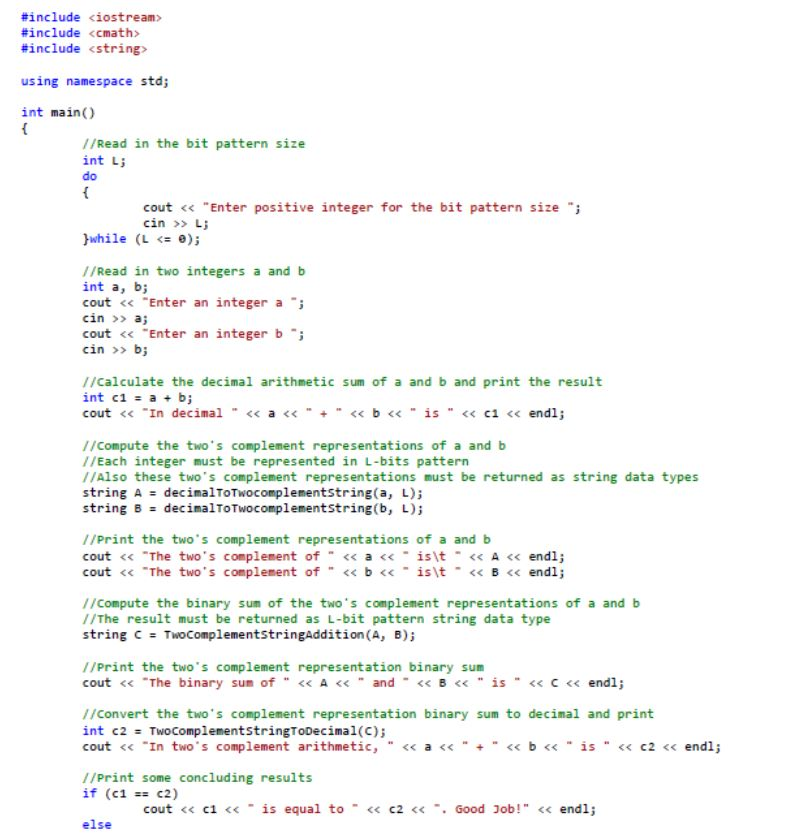2018-07-06 03:48

# c++针对二进制补码算术中整数的算术运算问题，请大神指点

c++ 二进制问题请教

01001和10010的二进制和是11011。

#include iostream>
#include cmath>
#include string>

using namespace std;

int main()
{
//Read in the bit pattern size
int L;
do {
cout << "Enter positive integer for the bit pattern size ";
cin >> L;
}while (L <= 0);

``````    //Read in two integers a and b
int a, b;
cout << "Enter an integer a ";
cin >> a;
cout << "Enter an integer b ";
cin >> b;

//Calculate the decimal arithmetic sum of a and b and print the result int
c1 = a + b;
cout << "In decimal " << a << " + " << b << " is " << c1 << endl;

//Compute the two's complement representations of a and b
//Each integer must be represented in L-bits pattern
//Also these two's complement representations must be returned as string data types
string A = decimalToTwocomplementString(a, L);
string B = decimalToTwocomplementString(b, L);

//Print the two's complement representations of a and b
cout << "The two's complement of " << a << " is\t " << A << endl;
cout << "The two's complement of " << b << " is\t " << B << endl;

//Compute the binary sum of the two's complement representations of a and b
//The result must be returned as L-bit pattern string data type
string C = TwoComplementStringAddition(A, B);

//Print the two's complement representation binary sum
cout << "The binary sum of " << A << " and " << B << " is " << C << endl;

//Convert the two's complement representation binary sum to decimal and print
int c2 = TwoComplementStringToDecimal(C);
cout << "In two's complement arithmetic, " << a << " + " << b << " is " << c2 << endl;

//Print some concluding results
if (c1 == c2) cout << c1 << " is equal to " << c2 << ". Good Job!" << endl;
else
``````

{
cout << c1 << " is not equal to " << c2 << endl;
cout << "Either " << c1 << " cannot be represented by the given bit pattern OR we have made some mistake!" << endl;
}
system("Pause");
return 0;
}